# SAT Math Multiple Choice Question 101: Answer and Explanation

### Test Information

Question: 101

11.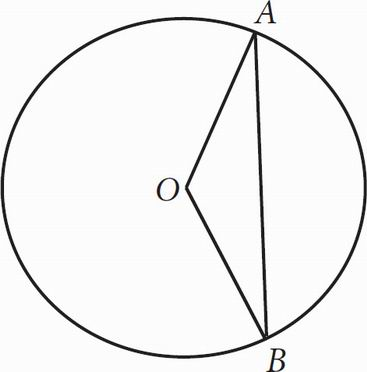If the radius of the circle above is x,AOB = 120°, and O is the center of the circle, what is the length of chord AB in terms of x ?

• A.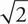x
• B.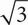x
• C.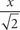• D.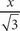B You can start by Plugging In a value for x; try x = 4. Because angle AOB is 120° and the triangle is isosceles, angles A and B are each 30°. Cut triangle AOB in half to make two 30-60-90 triangles with a hypotenuse of 4 and sides of 2 and 2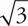. The side with length 2lies on chord AB. Double it to get the total length: 4or justx, which is (B) when you put x = 4 into the answer choices.